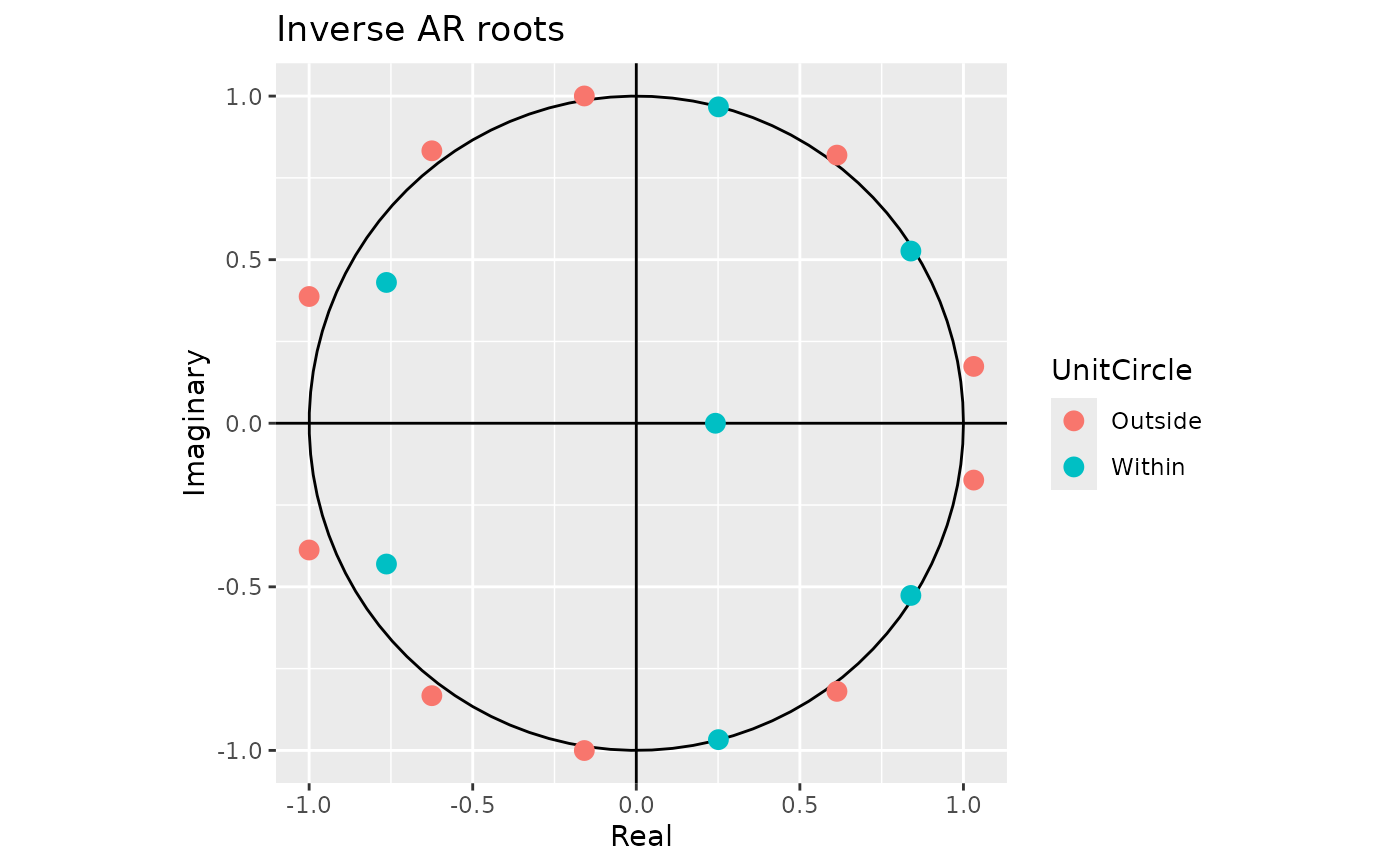Produces a plot of the inverse AR and MA roots of an ARIMA model. Inverse roots outside the unit circle are shown in red.

# S3 method for Arima
plot(
x,
type = c("both", "ar", "ma"),
main,
xlab = "Real",
ylab = "Imaginary",
...
)

# S3 method for ar
plot(x, main, xlab = "Real", ylab = "Imaginary", ...)

# S3 method for Arima
autoplot(object, type = c("both", "ar", "ma"), ...)

# S3 method for ar
autoplot(object, ...)

## Arguments

x Object of class “Arima” or “ar”. Determines if both AR and MA roots are plotted, of if just one set is plotted. Main title. Default is "Inverse AR roots" or "Inverse MA roots". X-axis label. Y-axis label. Other plotting parameters passed to par. Object of class “Arima” or “ar”. Used for ggplot graphics (S3 method consistency).

## Value

None. Function produces a plot

## Details

autoplot will produce an equivalent plot as a ggplot object.

Arima, ar

## Author

Rob J Hyndman & Mitchell O'Hara-Wild

## Examples


library(ggplot2)

fit <- Arima(WWWusage, order = c(3, 1, 0))
plot(fit)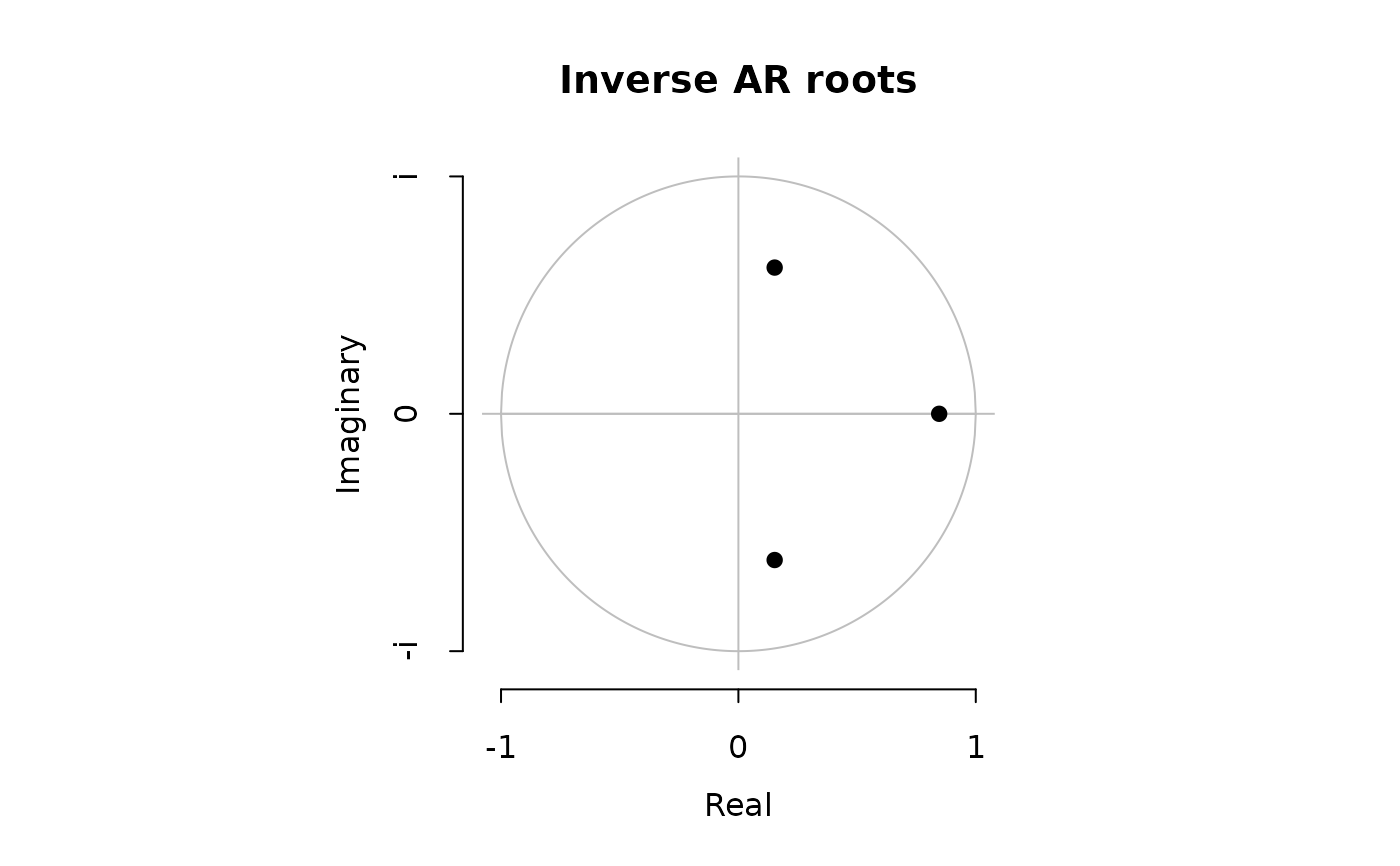autoplot(fit)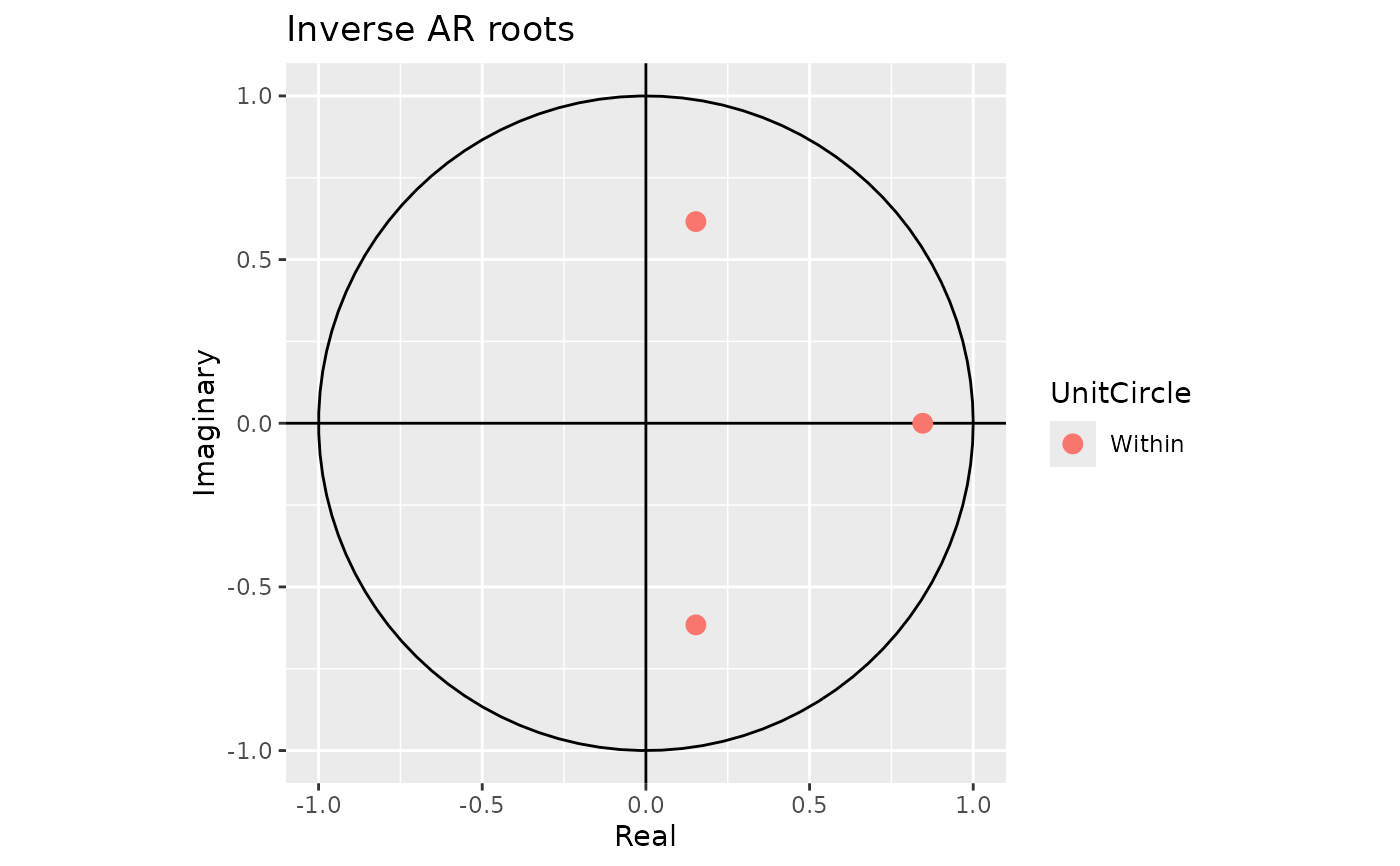fit <- Arima(woolyrnq, order = c(2, 0, 0), seasonal = c(2, 1, 1))
plot(fit)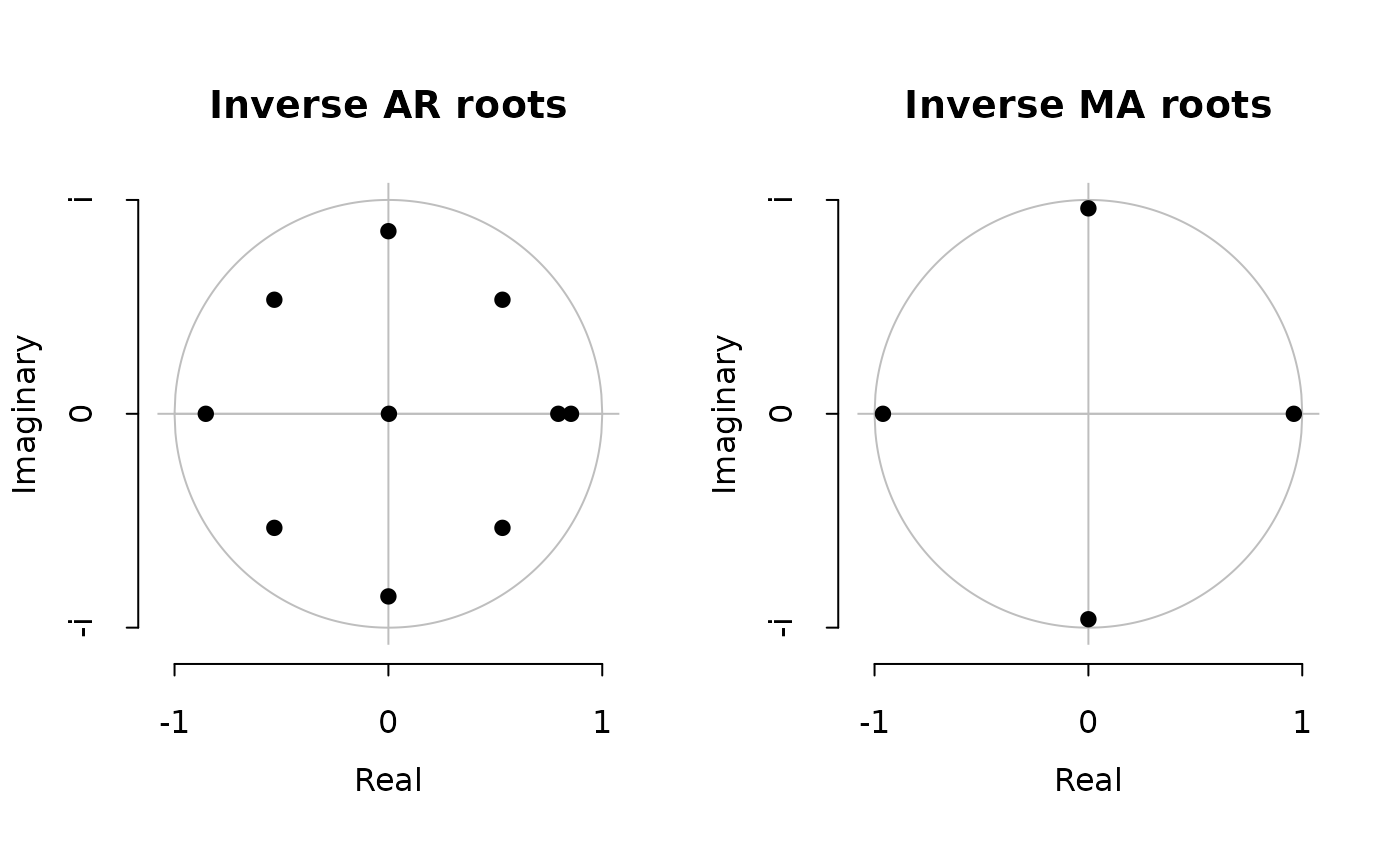autoplot(fit)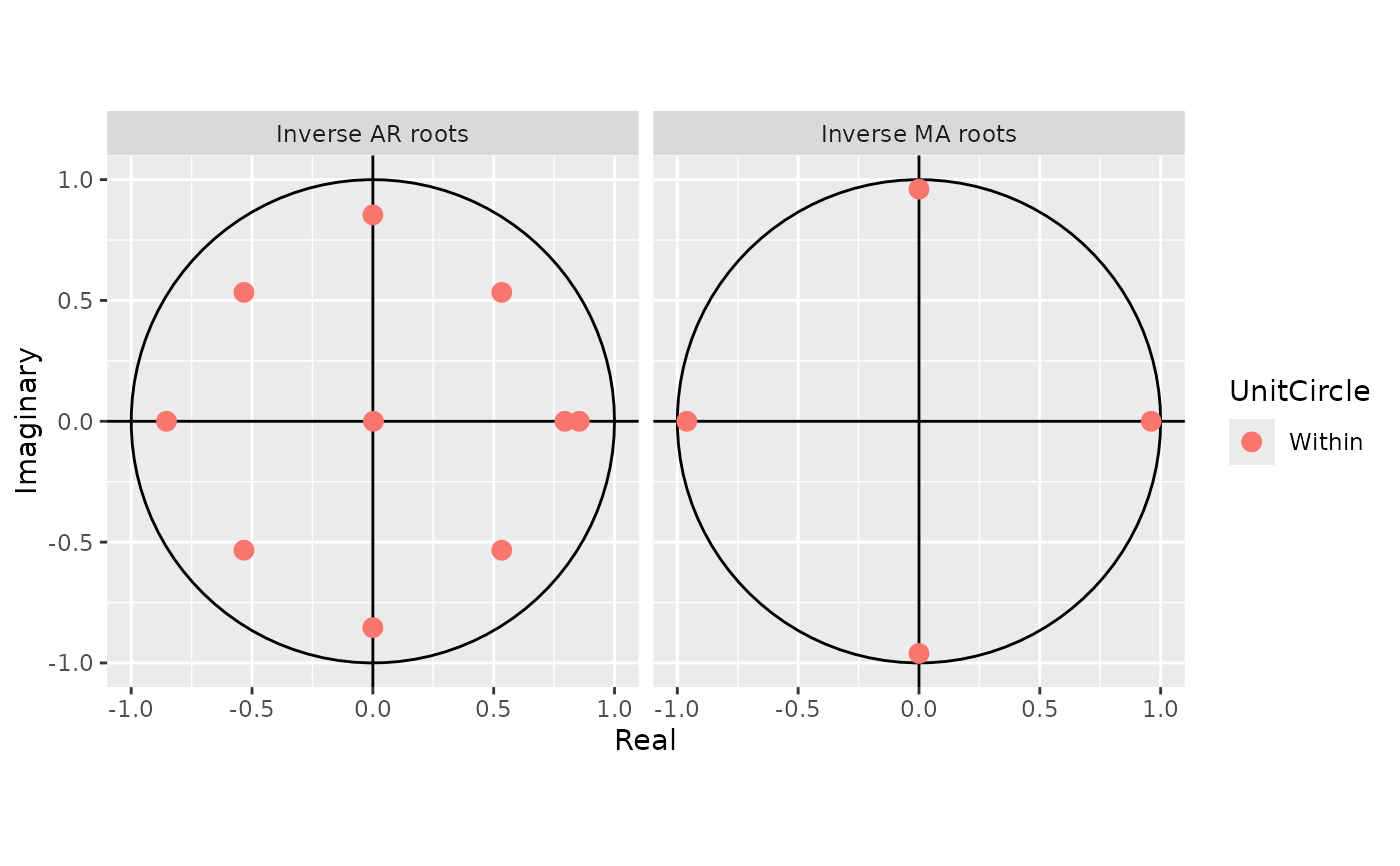plot(ar.ols(gold[1:61]))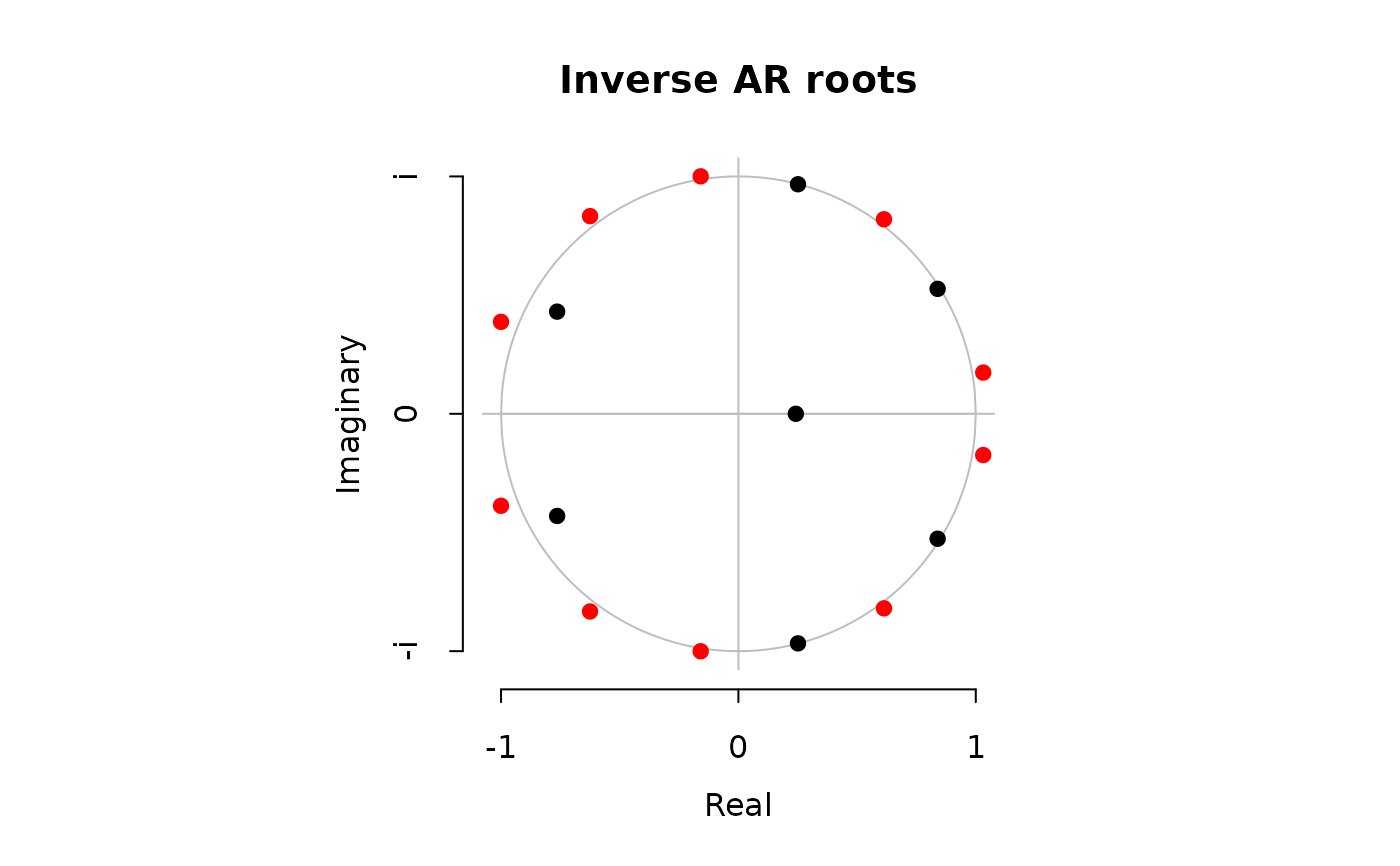autoplot(ar.ols(gold[1:61]))